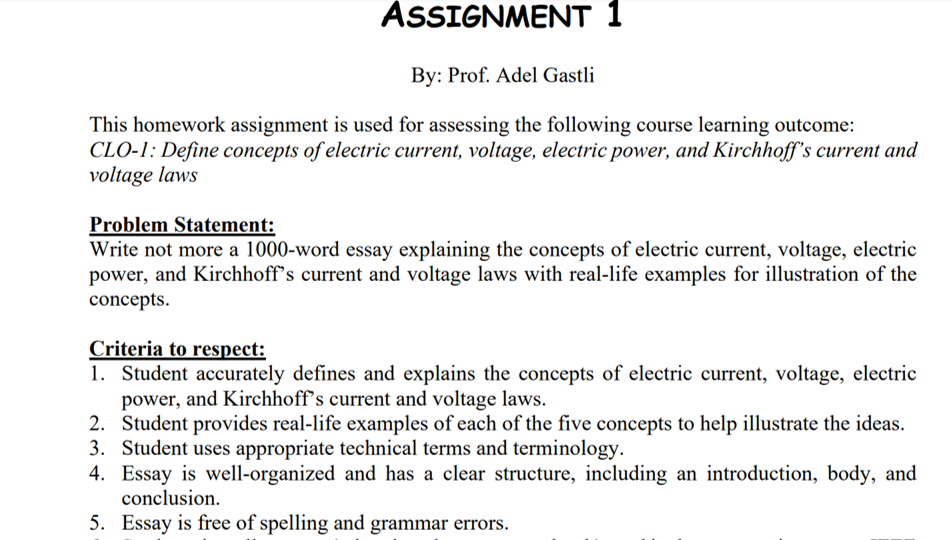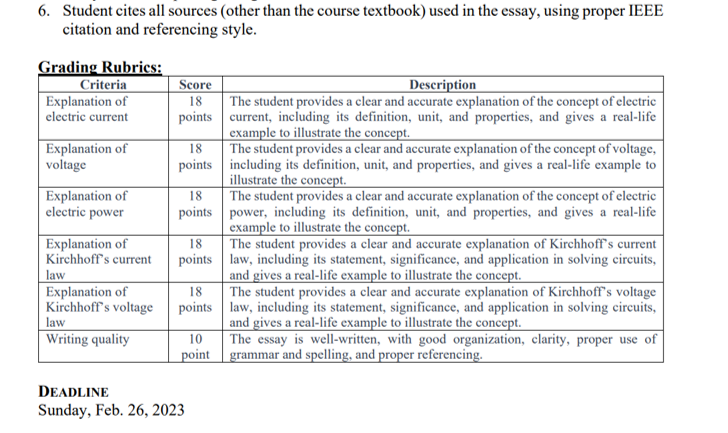# Question   please write them in text; and don't write less than 1000 word    I need it within  3  hours      This homework assignment is used for assessing the following course learning outcome: CLO-1: Define concepts of electric current, voltage, electric power, and Kirchhoff's current and voltage laws Problem Statement: Write not more a 1000 -word essay explaining the concepts of electric current, voltage, electric power, and Kirchhoff's current and voltage laws with real-life examples for illustration of the concepts. Criteria to respect: 1. Student accurately defines and explains the concepts of electric current, voltage, electric power, and Kirchhoff's current and voltage laws. 2. Student provides real-life examples of each of the five concepts to help illustrate the ideas. 3. Student uses appropriate technical terms and terminology. 4. Essay is well-organized and has a clear structure, including an introduction, body, and conclusion. 5. Essay is free of spelling and grammar errors. 6. Student cites all sources (other than the course textbook) used in the essay, using proper IEEE citation and referencing style. n $$\cdots \cdots \cdot$$ DEADLINE Sunday, Feb. 26, 2023WYSURD The Asker · Electrical Engineering

please write them in text; and don't write less than 1000 word

I need it within  3  hoursTranscribed Image Text: This homework assignment is used for assessing the following course learning outcome: CLO-1: Define concepts of electric current, voltage, electric power, and Kirchhoff's current and voltage laws Problem Statement: Write not more a 1000 -word essay explaining the concepts of electric current, voltage, electric power, and Kirchhoff's current and voltage laws with real-life examples for illustration of the concepts. Criteria to respect: 1. Student accurately defines and explains the concepts of electric current, voltage, electric power, and Kirchhoff's current and voltage laws. 2. Student provides real-life examples of each of the five concepts to help illustrate the ideas. 3. Student uses appropriate technical terms and terminology. 4. Essay is well-organized and has a clear structure, including an introduction, body, and conclusion. 5. Essay is free of spelling and grammar errors. 6. Student cites all sources (other than the course textbook) used in the essay, using proper IEEE citation and referencing style. n $$\cdots \cdots \cdot$$ DEADLINE Sunday, Feb. 26, 2023
More
Transcribed Image Text: This homework assignment is used for assessing the following course learning outcome: CLO-1: Define concepts of electric current, voltage, electric power, and Kirchhoff's current and voltage laws Problem Statement: Write not more a 1000 -word essay explaining the concepts of electric current, voltage, electric power, and Kirchhoff's current and voltage laws with real-life examples for illustration of the concepts. Criteria to respect: 1. Student accurately defines and explains the concepts of electric current, voltage, electric power, and Kirchhoff's current and voltage laws. 2. Student provides real-life examples of each of the five concepts to help illustrate the ideas. 3. Student uses appropriate technical terms and terminology. 4. Essay is well-organized and has a clear structure, including an introduction, body, and conclusion. 5. Essay is free of spelling and grammar errors. 6. Student cites all sources (other than the course textbook) used in the essay, using proper IEEE citation and referencing style. n $$\cdots \cdots \cdot$$ DEADLINE Sunday, Feb. 26, 2023